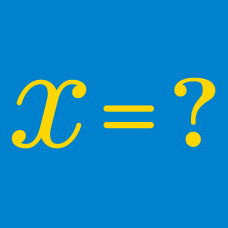Algebra

# Algebra Warmups: Level 4 Challenges

Given that

$\frac{ \color{#D61F06}{a}}{\color{#3D99F6}{b}+\color{#EC7300}{c}} + \frac{\color{#3D99F6}{b}} {\color{#D61F06}{a}+\color{#EC7300}{c}} + \frac{ \color{#EC7300}{c}}{\color{#D61F06}{a}+\color{#3D99F6}{b}} = 1,$

find the value of

$\large \frac{ \color{#D61F06}{a}^2}{\color{#3D99F6}{b}+\color{#EC7300}{c}} + \frac{ \color{#3D99F6}{b}^2}{\color{#D61F06}{a}+\color{#EC7300}{c}} + \frac{ \color{#EC7300}{c}^2} { \color{#D61F06}{a}+\color{#3D99F6}{b}}.$

Find the sum of all solutions to the equation

$\large (x^2+5x+5)^{x^2-10x+21}=1 .$

A polynomial $f(x)$ satisfies the equation $f(x)+(x+1)^3=2f(x+1)$. Find $f(10)$.

Let $f(x)$ be a quintic polynomial such that

$\begin{array} { r l } f(1) & = 1 \\ f(2) & = 1 \\ f(3) & = 2 \\ f(4) & = 3 \\ f(5) & = 5 \\ f(6) & = 8. \\ \end{array}$

Determine $f(7)$.


Note: Many people are answering this incorrectly because they think it is the Fibonacci sequence, but this problem is asking about a quintic polynomial that passes through those points. That does not necessarily mean the next term behaves as the Fibonacci sequence would.

\begin{aligned} |a - b | &=& 2 \\ |b - c | &=& 3 \\ |c - d | &=& 4 \\ \end{aligned}

Given that $a,b,c,d$ are real numbers that satisfy the system of equations above, what is the sum of all distinct values of $|a-d|$?

×

Problem Loading...

Note Loading...

Set Loading...